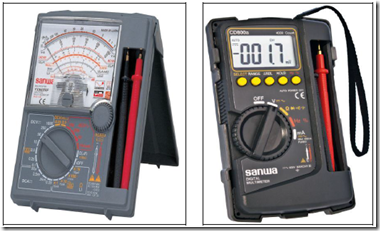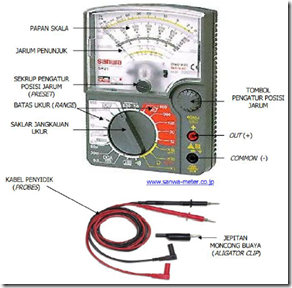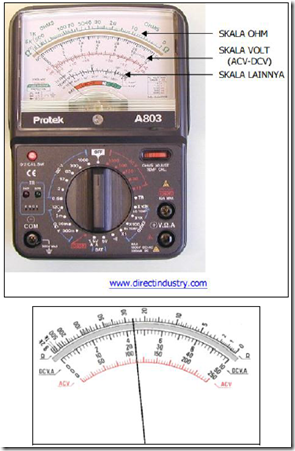" /> Configuring Multimeter - TN Elektro
Home > English > Configuring Multimeter

# Configuring Multimeter

Multimeter described in this book is Multimeter Analog that use rotating coils to move the needle board scale. Multimeter is widely used because they are relatively affordable. If the Digital Multimeter direct measurement results can be read in the form of numbers that appear on the screen display, the analog multimeter measurement results read by the appointment of a needle on the scale board. See figure 1 and figure 2.Figure 1. Figure 2. Analog Multimeter Digital Multimeter

Multimeter configuration and control indicators contained in a Multimeter shown in Figure 3.Figure 3. Configuration Multimeter

a. board Scale
Used to read the measurement results. On board there is a scale of scales; custody / resistance (resistance) in units of Ohm (Ω), voltage (ACV and DCV), strong currents (DCMA), and other scales. See Figure 4.Figure 4. Board Scale

b. Reach switch Measure
Measure Range switch: used to determine the position of the working Multimeter, and the measuring limit (range). If the unit used to measure the value of resistance (in Ω), the switch is placed in the position Ω, likewise if it is used to measure the voltage (ACV-DCV), and a strong current (mA-uA). One thing to remember, in measuring voltage, the switch position should be in the range of measuring higher than the voltage to be measured. For example, the voltage to be measured 220 ACV, the switch must be in the position measuring limit 250 ACV. Likewise, if you want to measure DCV.

c. Regulatory screw Needle position (preset)
Used to menera needle at zero (left board scale).

d. Regulatory button Needle In Position Zero (Zero Adjustment)
Used to menera needle on zero before Multimeter used to measure the value of resistance / resistance. In practice, both ends of the investigator (probes) met, the key is rotated to position the needle at zero.

e. Cable holes Investigators
A place to connect the wires investigator with Multimeter. Marked with a (+) or out and (-) or common. In a more complete Multimeter there is also a hole to measure hfe transistor (strengthening of the direct current / DCMA by transistors based on the functions and types), and the hole to measure the capacity of capacitors.